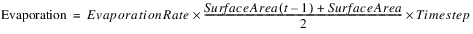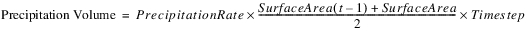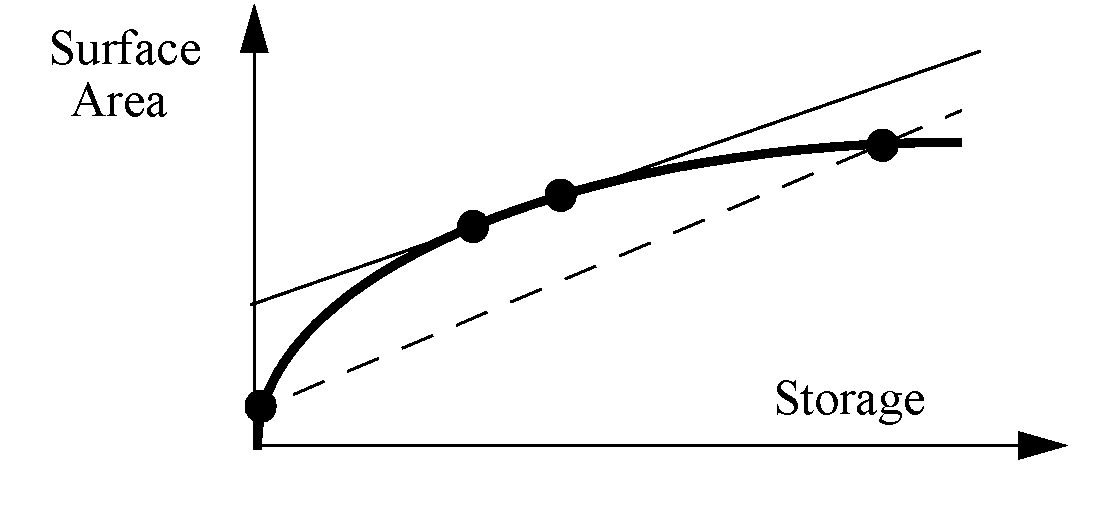Optimization Evaporation
This category can be used to model evaporation and precipitation.None
This is the default method for this category. It does no calculations, and it requires that None be selected for the Evaporation and Precipitation category.Opt Input Evaporation
This method is analogous to the Input Evaporation method in simulation, and it requires the Input Evaporation method to be selected for the Evaporation and Precipitation category. Evaporation Rate and Precipitation Rate are entered as a time series. Evaporation is calculated as a product of Evaporation Rate, Average Surface Area over the timestep and Timestep length. Similarly Precipitation Volume is calculated as the product of Precipitation Rate, Average Surface Area and Timestep length.
Evaporation and Precipitation are calculated based on a static Volume-Surface Area relationship. In reality the Volume-Surface Area relationship may not be static for a slope storage reservoir, so results from the Opt Input Evaporation method should be treated with caution when used on a slope power reservoir object.
Caution:  The linearization of the Surface Area variable can result in a small approximation error in optimization for Evaporation and Precipitation Volume. This means there can be a small difference between the mass balances in the optimization solution and the post-optimization rulebased simulation when using this method. It is important to use care when setting the approximation points for Surface Area in order to reduce this approximation error. Refer to the information on the Surface Area LP Param slot below. Also caution should be used if applying this method at a 1 Month timestep. All rates in the optimization mass balance are converted to monthly volumes based on a 30-day month, regardless of the month. This will also produce a difference between the mass balances in the optimization solution and the post-optimization rulebased simulation for a 1 Month timestep.
Slots Specific to This Method
Elevation Area Table
Type: Table Slot
Units: Length vs Area
Description: Represents the Elevation-Surface Area relationship
Information: This table must be input. It is used to derive the Volume Area Table.
Defined by: User input
Evaporation
Type: Series Slot
Units: Volume
Description: The volume of water lost to evaporation over the timestep
Information: If this slot contains user input, it is added directly to the mass balance constraint, otherwise it is defined by the expression below.
Defined by: Either user input or the following constraint:Evaporation Rate
Type: Series Slot
Units: Velocity
Description: The rate, in length per time, at which water is lost to evaporation at each timestep
Information: This slot can be set as user input. If it is not set as an input, and if Evaporation is not an input, this slot defaults to zero. If Evaporation is an input, this slot is not used.
Defined by: User input or defaults to zero
Precipitation Rate
Type: Series Slot
Units: Velocity
Description: The rate, in length per time, at which water is gained from precipitation at each timestep
Information: This slot can be set as user input. If it is not set as an input, it defaults to zero.
Defined by: User input or defaults to zero
Precipitation Volume
Type: Series Slot
Units: Volume
Description: The volume of water gained from precipitation over the timestep
Information: The Input Evaporation method will not allow this slot to be set as an input.
Defined by: Explicit constraint:Surface Area
Type: Series Slot
Units: Area
Description: The area of the water surface at the end of the timestep
Information: This slot is numerically approximated as a function of Storage (Numerical 2-D Approximation). The relationship between Pool Elevation and Storage comes from the automatically generated Volume Area Table. The table is queried using the user-input points defined in the Surface Area LP Param table.
Defined by: Numerical 2-D Approximation in terms of Storage, based upon the Volume Area Table. The Surface Area LP Param table values are used as approximation points indexing the Volume Area Table. The preferred order of approximation is substitution, piecewise, two-point line, tangent. Most often the two-point line (secant) approximation will be used.
Surface Area LP Param
Type: Table Slot
Units: Volume
Description: Specifies the Storage points used to take the tangent, line and piecewise approximations for Surface Area linearization.
Information: The best Storage point to choose for tangent approximation would be the expected storage during the run; for line approximation, the expected maximum and minimum Storage; for piecewise approximation, use points that cover the full range of expected Storage during the run with intermediate points such that a piecewise linear curve reasonably approximates the actual curve. In most cases, the line (secant) approximation will be used. It is important to set these points carefully to minimize approximation error.
Defined by: User input
The following figure represents two alternative selections of points for the line approximation in the Surface Area LP Param table. The linear approximation represented by the dashed line corresponds to the selection of points near the extremes of the Volume Area table. This approximation will tend to result in an under-estimation of Surface Area, and thus an under-estimation of Evaporation and Precipitation Volume. Evaporation losses in the post-optimization rulebased simulation would be greater than the losses approximated in the optimization solution. The linear approximation represented by the solid line corresponds to the selection of points closer together in the Volume Area Table, and is more similar to the Tangent approximation. This approximation would tend to result in an over estimation of Surface Area, and the losses due to Evaporation in the post-optimization rulebased simulation would be less than the approximation in the optimization solution.
Surface area vs storage relationship with two alternative line approximations. Not drawn to scale.Volume Area Table
Type: Table Slot
Units: Volume vs Area
Description: Represents the Storage Volume-Surface Area relationship
Information: This table is read-only and is automatically generated at the start of the run from the Elevation Area Table and the Elevation Volume table. The method starts by copying the Elevation Area Table and then replaces the Pool Elevation Values with the corresponding Storage values. The Storage values are linearly interpolated based on the Elevation Volume Table
Defined by: Automatically generated
Revised: 08/02/2021